Examples

Probability
Serial order wise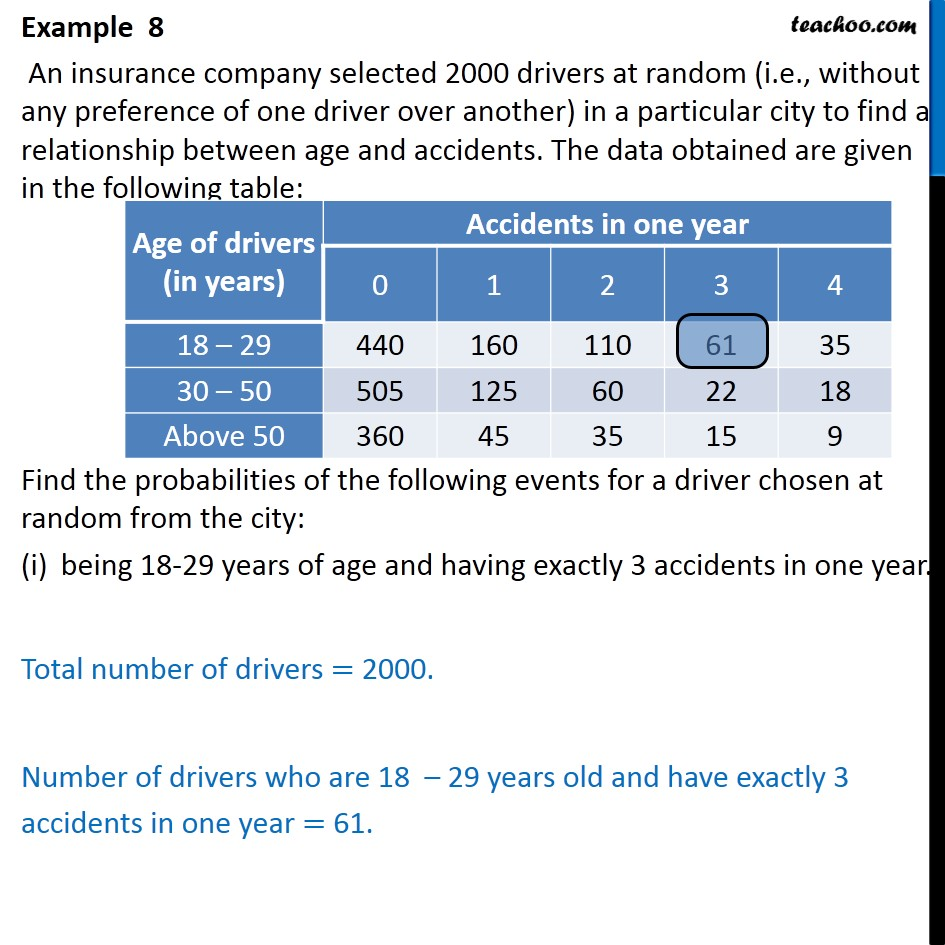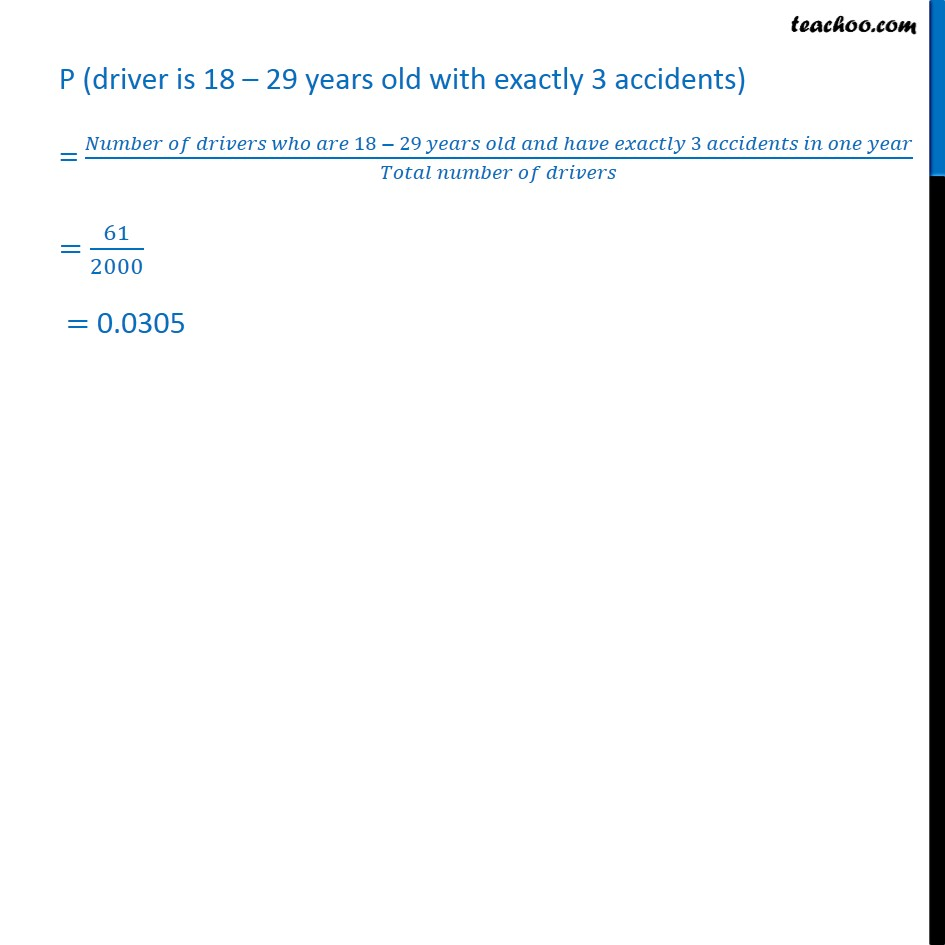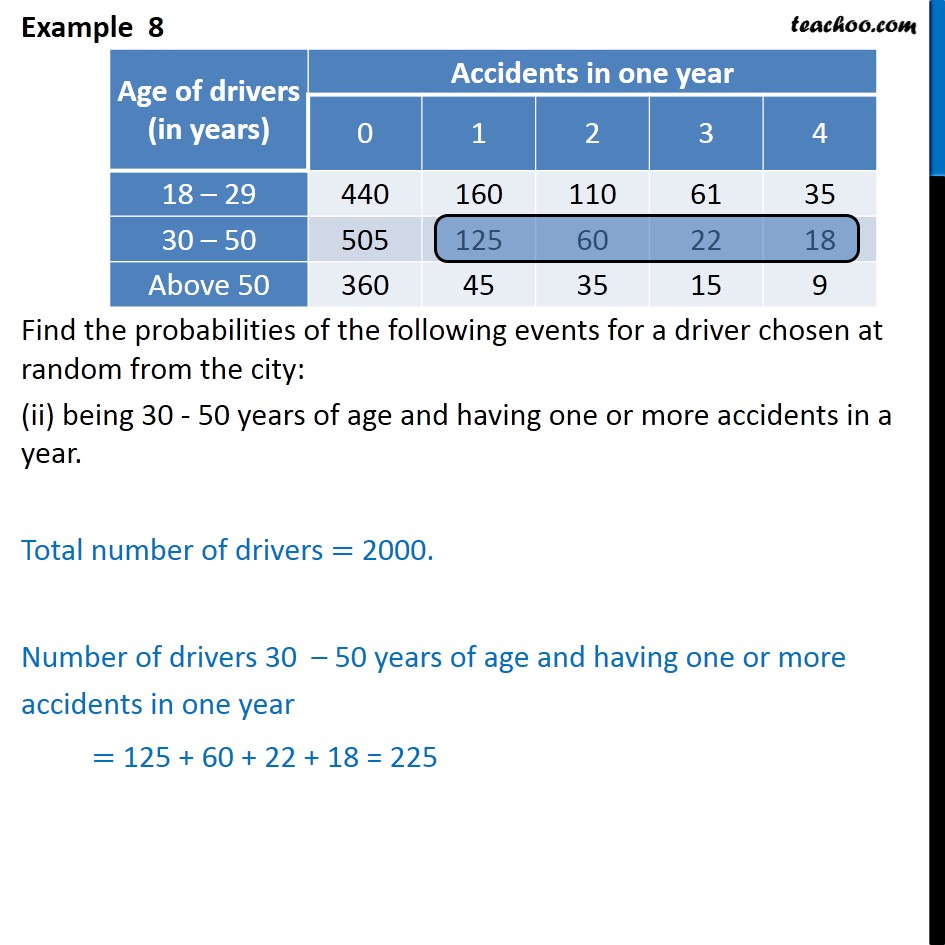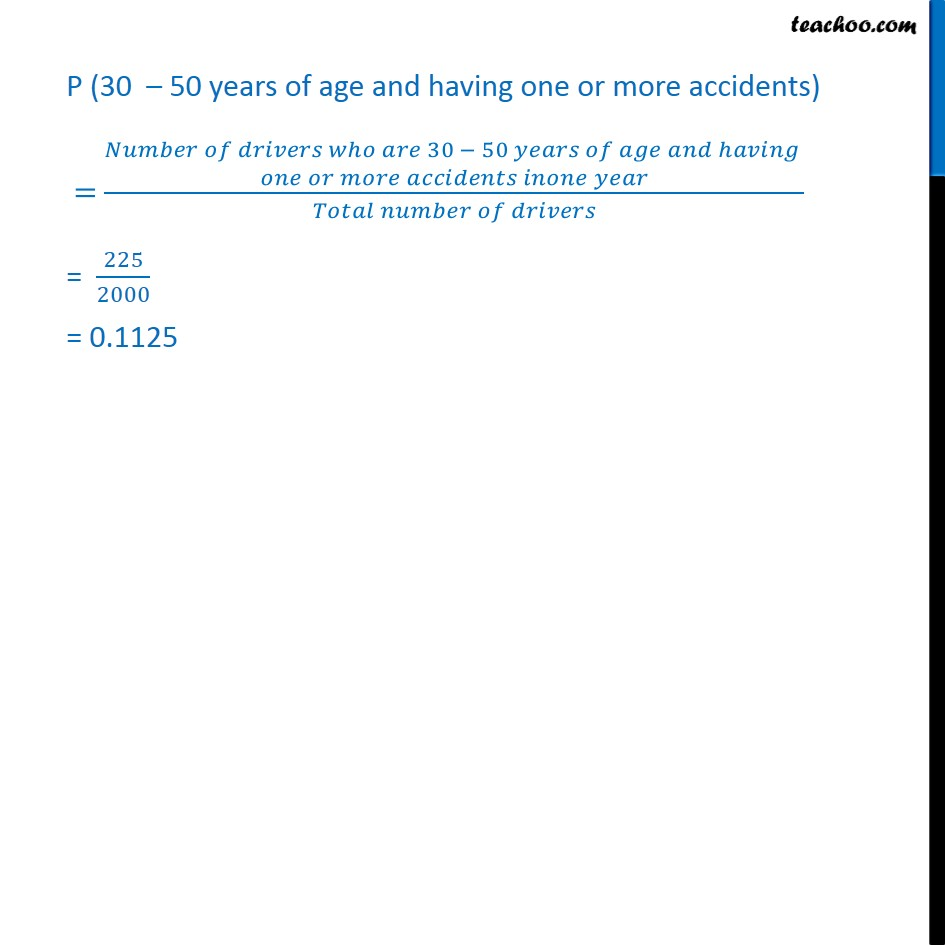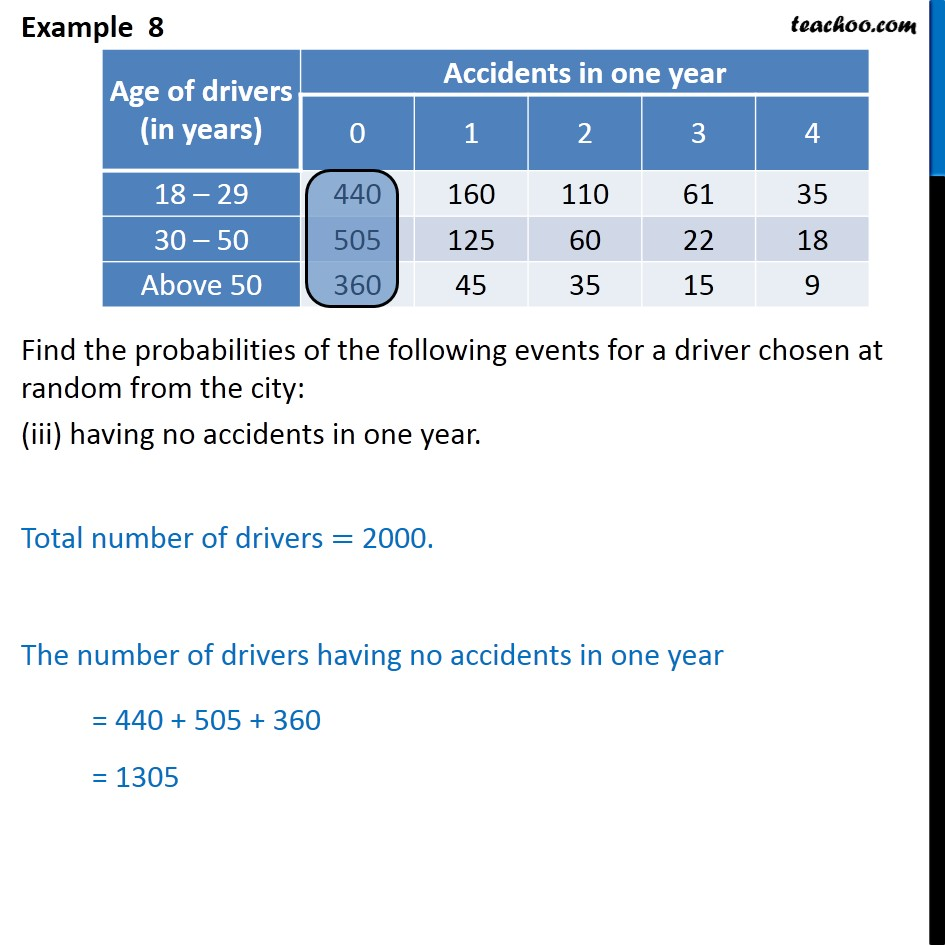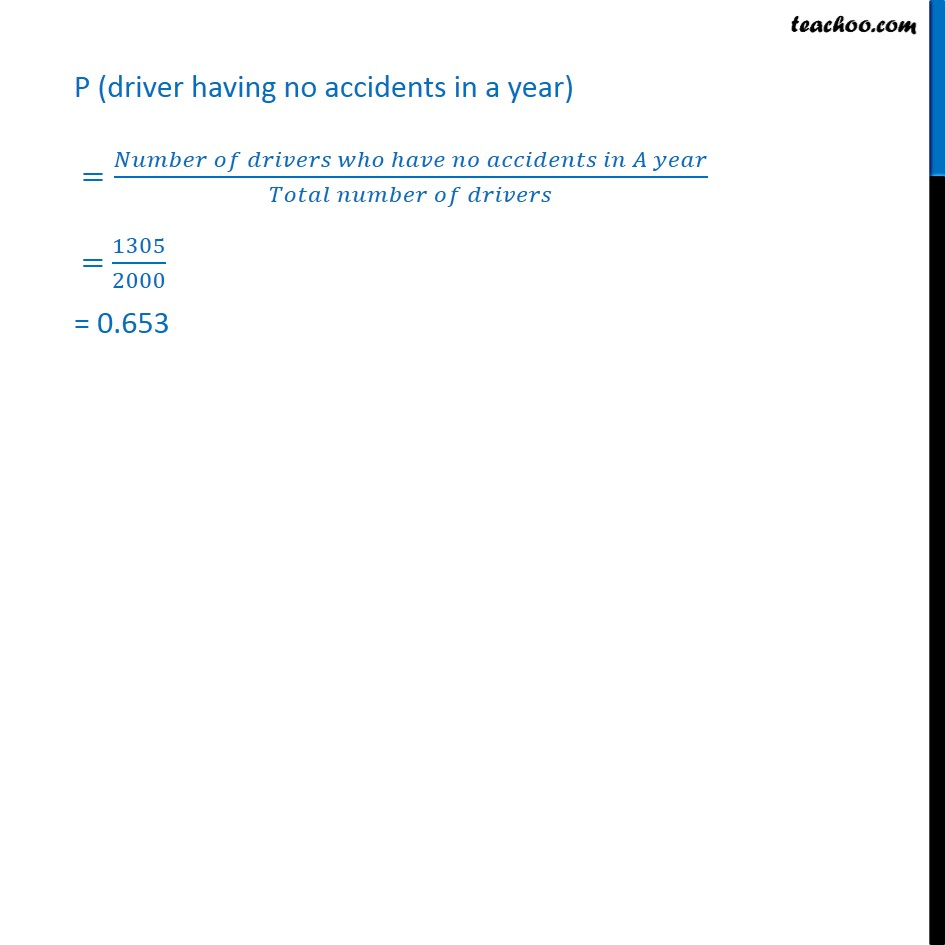Learn in your speed, with individual attention - Teachoo Maths 1-on-1 Class

### Transcript

Example 8 An insurance company selected 2000 drivers at random (i.e., without any preference of one driver over another) in a particular city to find a relationship between age and accidents. The data obtained are given in the following table: Find the probabilities of the following events for a driver chosen at random from the city: being 18-29 years of age and having exactly 3 accidents in one year. Total number of drivers = 2000. Number of drivers who are 18 – 29 years old and have exactly 3 accidents in one year = 61. P (driver is 18 – 29 years old with exactly 3 accidents) = (𝑁𝑢𝑚𝑏𝑒𝑟 𝑜𝑓 𝑑𝑟𝑖𝑣𝑒𝑟𝑠 𝑤ℎ𝑜 𝑎𝑟𝑒 18 − 29 𝑦𝑒𝑎𝑟𝑠 𝑜𝑙𝑑 𝑎𝑛𝑑 ℎ𝑎𝑣𝑒 𝑒𝑥𝑎𝑐𝑡𝑙𝑦 3 𝑎𝑐𝑐𝑖𝑑𝑒𝑛𝑡𝑠 𝑖𝑛 𝑜𝑛𝑒 𝑦𝑒𝑎𝑟)/(𝑇𝑜𝑡𝑎𝑙 𝑛𝑢𝑚𝑏𝑒𝑟 𝑜𝑓 𝑑𝑟𝑖𝑣𝑒𝑟𝑠) = 61/2000 = 0.305 Example 8 Find the probabilities of the following events for a driver chosen at random from the city: (ii) being 30 - 50 years of age and having one or more accidents in a year. Total number of drivers = 2000. Number of drivers 30 – 50 years of age and having one or more accidents in one year = 125 + 60 + 22 + 18 = 225 P (30 – 50 years of age and having one or more accidents) = █(𝑁𝑢𝑚𝑏𝑒𝑟 𝑜𝑓 𝑑𝑟𝑖𝑣𝑒𝑟𝑠 𝑤ℎ𝑜 𝑎𝑟𝑒 30 − 50 𝑦𝑒𝑎𝑟𝑠 𝑜𝑓 𝑎𝑔𝑒 𝑎𝑛𝑑 ℎ𝑎𝑣𝑖𝑛𝑔 @𝑜𝑛𝑒 𝑜𝑟 𝑚𝑜𝑟𝑒 𝑎𝑐𝑐𝑖𝑑𝑒𝑛𝑡𝑠 𝑖𝑛𝑜𝑛𝑒 𝑦𝑒𝑎𝑟)/(𝑇𝑜𝑡𝑎𝑙 𝑛𝑢𝑚𝑏𝑒𝑟 𝑜𝑓 𝑑𝑟𝑖𝑣𝑒𝑟𝑠) = 225/2000 = 0.1125 Example 8 Find the probabilities of the following events for a driver chosen at random from the city: (iii) having no accidents in one year. Total number of drivers = 2000. The number of drivers having no accidents in one year = 440 + 505 + 360 = 1305 P (driver having no accidents in a year) =(𝑁𝑢𝑚𝑏𝑒𝑟 𝑜𝑓 𝑑𝑟𝑖𝑣𝑒𝑟𝑠 𝑤ℎ𝑜 ℎ𝑎𝑣𝑒 𝑛𝑜 𝑎𝑐𝑐𝑖𝑑𝑒𝑛𝑡𝑠 𝑖𝑛 𝐴 𝑦𝑒𝑎𝑟)/(𝑇𝑜𝑡𝑎𝑙 𝑛𝑢𝑚𝑏𝑒𝑟 𝑜𝑓 𝑑𝑟𝑖𝑣𝑒𝑟𝑠) = 1305/2000 = 0.653